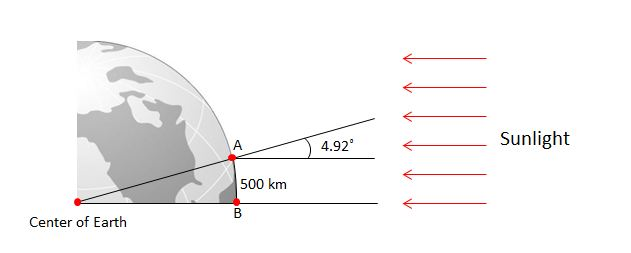# Circumference of Earth

Geometry Level 2At point B on the earth, it appears to someone standing on the surface that the sun's rays are coming straight down from the sun. At point A, it appears to someone standing on the surface that the sun's rays are coming in at an angle of $4.92^\circ$. If, the distance between $A$ and $B$ is $500$ km, traveling along the earth's surface, how long is the circumference of the earth?

(Assume that earth is perfect sphere and that rays from the sun are effectively all coming towards the earth parallel to each other.)

×